## Introduction to Javascript void

Void means completely emptyIn JavaScript, the void is an operator, which is used when the function is not return anything. It means the void result is undefined. In Java, if we do not specify any return type then automatically that function or method becomes void. But, JavaScript is not typed checking language. So, the function always returns something. In some situations we do not need to return anything so, we must specify void operator in front of the function.

Real-time Example: Overcome side effects while inserting any expressions into a web page, we might have used JavaScript:void(0).

### How does void operator work in JavaScript?

Not returning function in JavaScript always works with a void operator.

Syntax:

```void function functionName() { //code }```

Above syntax inside implementation:

```function functionName() { return undefined; }```

While not refresh and immovable links from page to page simply use void operator. This is always used with links.

Syntax:

`<a href="JavaScript:void(0);"/>`

### Examples

Here are the following examples mention below

#### Example #1

Void operator with function(without parameters)

```<!DOCTYPE html> <html> <head> <title>Void</title> </head> <body> <font color="green"> <h1>Void operator with function Even numbers</h1> </font> <script> void function getEvenNumbers() { document.write("Even numbers from 1 to 10=><br>"); for(let i=0;i<=10;i++) { if(i%2==0&&i!=0) document.write(i+"<br>") } }(); </script> </body> </html>```

Output: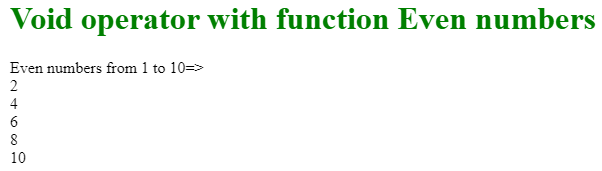Explanation:

As we can see above function do not have any chance to return anything, as it is a void function.

#### Example #2

The void operator with function(with parameters)

```<!DOCTYPE html> <html> <head> <title>Void</title> </head> <body> <font color="green"> <h1>Void operator with function Rectangle Area</h1> </font> <script> void function getAreaOfRectangle(length,breadth) { var area=length*breadth; document.write(length+": length and "+ breadth+" : breadth of area is ="+area); }(20,25); </script> </body> </html>```

Output: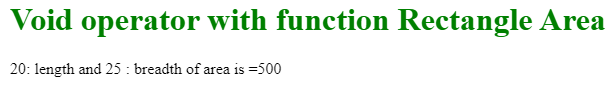Explanation:

As we can see the above code even passing with arguments also function not return anything because of the void operator.

#### Example #3

Void operator with numbers, VoidWithNumbers.js

```<!DOCTYPE html> <html> <head> <title>Void</title> </head> <body> <font color="green"> <h1>Void operator with Numbers</h1> </font> <script> function getNumbers(x,y,z) { x=void(y,z); document.write("x value : "+x+"<br> y value : "+y+"<br> z value: "+z); } getNumbers(1,2,3); </script> </body> </html>```

Output: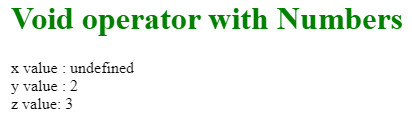Explanation

• As we can see y and z values are not changed because the resultant of y and z are not void.
• Whereas, x has resultant of the void operator with y and z. So, the result of x will become undefined.
• It proves void resultant always undefined primitive value.

#### Example #4

JavaScript:void(0)

```<!DOCTYPE html> <html> <head> <title>Void</title> </head> <body> <font color="green"> <h1>javascript void(0)</h1> </font> <h2> <a href="javascript:void(0);"> Void Link(It is not responding at all) </a> </h2> <h2> <a href="javascript:void(alert('Hello, I am void link with non zero'));"> Void Link(It is responding by popup alert box) </a> </h2> </body> </html>```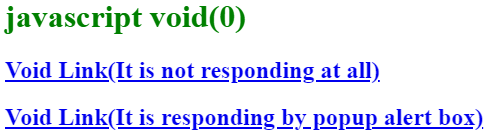Output after pressing the second link: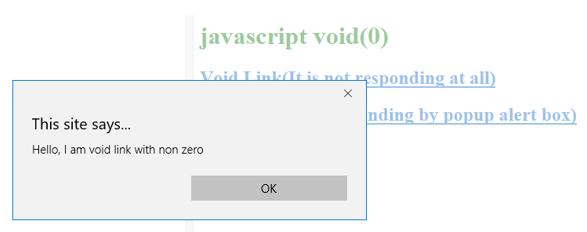Explanation

• When we press javascript:void(0) link(1st link), it does not work at all because it returns void(0)